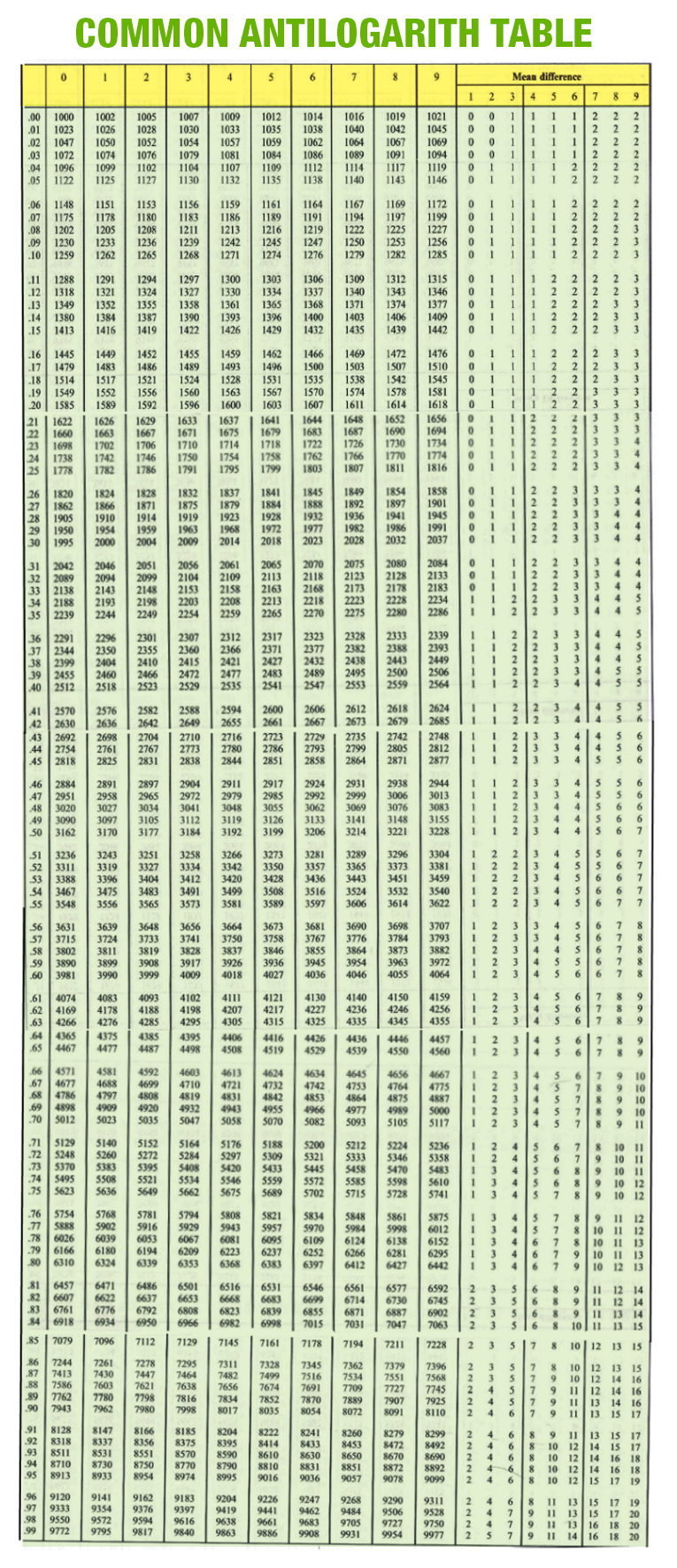# Antilog Table

Antilog Definition: The Antilog, which is also known as “Anti- Logarithms” of a number is the inverse technique of finding the logarithm of the same number. Consider, if x is the logarithm of a number y with base b, then we can say y is the antilog of x to the base b. It is defined by

 If logb y = x                           Then, y = antilog x

Both logarithm and antilog have their base as 2.7183. If the logarithm and antilogarithm have their base 10, that should be converted into natural logarithm and antilog by multiplying it by 2.303.

## How to Calculate Antilog?

Before finding the antilog of a number, we should know about the parts like the characteristic and mantissa part.

• Characteristic Part – The whole part is called the characteristic part. If the characteristic of logarithm of any number greater than one is positive and is one less than the number of digits in the left side of the decimal point.
• Mantissa Part – The decimal part of the logarithm number for a given number is called the mantissa part, and it should always be a positive value. If the mantissa part is in a negative value, convert into the positive value.

## Procedure to Find the Antilog of a Number

### Method 1: Using an Antilog Table

Consider a number, 2.6452

Step 1: Separate the characteristic part and the mantissa part. From the given example, the characteristic part is 2, and the mantissa part is 6452.

Step 2: To find a corresponding value of the mantissa part use the antilog table. Using the antilog table, find the corresponding value. Now, find the row number that starts with .64, then the column for 5. Now, you get the corresponding value as 4416.

Step 3: From mean difference columns find the value. Again use the same row number .64 and find the value for column 2. Now, the value corresponding to this is 2.

Step 4: Add the values obtained in step 2 and 3, we get 4416 + 2 = 4418.

Step 5: Now insert the decimal point. The decimal point always goes the designated place. For this, you have to add 1 to the characteristic value. Now you get 3. Then add the decimal point after 3 digits, we get 441.8

So the antilog value of 2.6452 is 441.8.

### Method 2: Antilog Calculation

Step 1: Separate the characteristic part and the mantissa part. From the above example given, the characteristic part is 2, and the mantissa part is 6452.

Step 2: Know the base. For numerical computations, the base is always 10. Therefore, for computing the antilog use base 10.

Step 3: Calculate the 10X. X is the number which you are using. If the mantissa of the number is 0, then the computation is easy. Calculate the value 102.6452. Use a calculator to find the value. Finally, it comes to 441.7

Both methods will give the same result.

## Common Antilog Table

Below table helps to find the values of Characteristic Part and Mantissa Part of the number.## Sample Example

Question:

Find the antilog of 3.3010

Solution:

Given, antilog (3.3010)

Step 1: Characteristics part = 3 and mantissa part = 3010

Step 2: Use the antilog table for the row.30, then the column for 1, you get 2000.

Step 3: Find the value from the mean difference column for the row .30 and column 0, it gives the value 0

Step 4: Add the values obtained in step 2 and 3 , 2000 + 0 = 2000.

Step 5: Now insert the decimal place. We know that the characteristic part is 3 and we have to add it with 1. Therefore, we get the value 4. Insert the decimal point after 4 places, and we get 2000.

Therefore, the solution of the antilog 3.3010 is 2000.

To know about antilog tables, register with BYJU’S to get a clear knowledge on solving problems in an easy and engaging way.

 Related Links Logarithm Formula Logarithmic Differentiation Natural Log Formula Logarithm Calculator